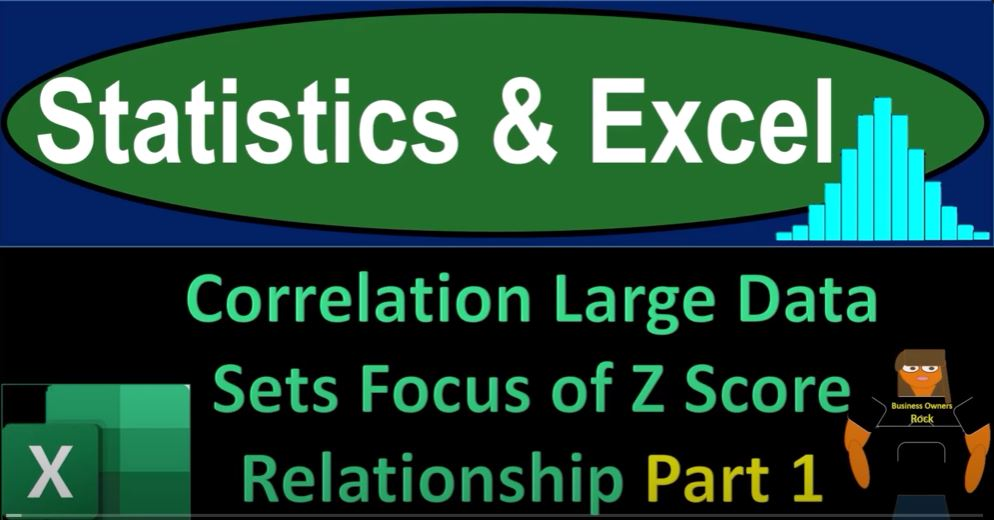## Correlation Large Data Sets Focus of Z Score Relationship Part 1 1750 Statistics & Excel

Welcome to part one of our journey into the world of statistics, Excel, and the fascinating realm of correlation analysis. In this blog post, we’re going to dive into the essential concepts of correlation, z-scores, and how they relate to large datasets. So, take a deep breath, hold it for 10 seconds, and let’s embark on this enlightening Excel adventure.

Getting Started with Excel

We’ll start by exploring the world of Excel, where we’ll be working with a substantial dataset. Don’t worry if you don’t have access to the exact workbook we’re using; you can replicate it from scratch. Our workbook contains three tabs: “Example,” “Practice,” and “Blank.”

• “Example” showcases different datasets and their correlation analysis.
• “Practice” tab provides pre-formatted cells for practice problems.
• “Blank” tab is where we’ll begin, with just the initial data.

If you don’t have the dataset, you can easily find sample datasets on platforms like Kaggle.com.

Understanding the Concept of Correlation

In this blog, we will be examining the correlation between two extensive datasets – heights and weights. These datasets look promising for correlation analysis, as one might assume that people who are taller tend to weigh more. This hints at a potential mathematical relationship between height and weight.

However, having datasets that follow a similar bell-shaped curve doesn’t necessarily imply a correlation. Similar curves can occur for unrelated reasons, as we’ve seen with uniform distributions and random number sets.

The Role of Z-Scores

To assess the correlation, we’ll utilize z-scores extensively. These scores are central to understanding the relationship between two datasets. By comparing the z-scores of heights and weights, we can gain a better insight into their correlation.

Let’s Start Calculating

In Excel, we’ll calculate the mean and standard deviation for both height and weight. This lays the foundation for our analysis. Here’s how you can calculate these in Excel:

• Mean for height: `=AVERAGE(range)`
• Mean for weight: `=AVERAGE(range)`
• Standard deviation for height: `=STDEVP(range)`
• Standard deviation for weight: `=STDEVP(range)`

Creating Bell Curves

Next, we’ll create bell curves to visualize our datasets. You can use Excel’s histogram chart for this purpose. This step helps us identify the shape and distribution of our data.

Z-Scores Calculation

The core of our correlation analysis lies in calculating z-scores. Z-scores tell us how many standard deviations a data point is from the mean. For heights (H) and weights (W), the formula for z-scores is:

`Z = (X - Mean) / Standard Deviation`

Now, we’ll multiply the z-scores of heights and weights to proceed with our correlation calculations.

Analyzing the Correlation

To calculate the correlation according to our formula, we’ll divide the sum of the products of z-scores by n-1 (where n is the total number of data points). Excel’s DATA ANALYSIS tool can also help with this calculation.

Our calculation should yield a correlation value between -1 and 1. A positive value suggests a positive correlation, while a negative value indicates a negative correlation. A value close to 0 implies a weak or no correlation.

Double-Checking with Excel’s Data Analysis Tool

As a double-check, you can use Excel’s DATA ANALYSIS tool to calculate the correlation. This not only validates your work but also allows you to work with larger datasets efficiently.

In Conclusion

Correlation analysis and z-scores are powerful tools to understand relationships between datasets. In part two of our blog series, we’ll delve deeper into the analysis of z-scores and how they provide insights into the correlation between our datasets. Stay tuned for more data-driven discoveries!

In our next installment, we’ll explore the details of z-scores, providing you with a deeper understanding of their role in correlation analysis. So, until then, keep your Excel skills sharp, and get ready to unravel the mysteries of large datasets!